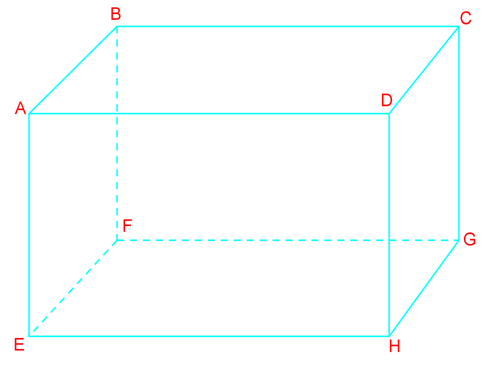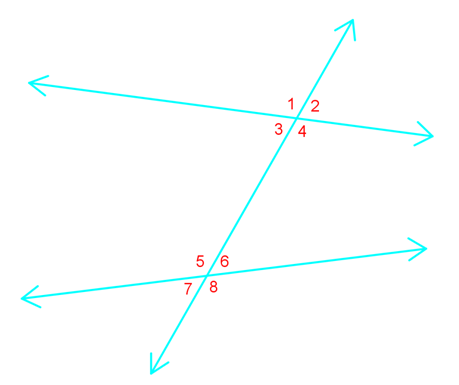# Pairs of lines and angles

### Pairs of lines and angles

#### Lessons

• Introduction
Relationships between lines and angles

• 1.
Below is a regular rectangular prism. Find.a)
Line(s) that are perpendicular to $\overline{AB}$.

b)
Line(s) that are parallel to $\overline{AB}$.

c)
Line(s) that are skew to $\overline{AB}$.

d)
Plane(s) parallel to plane $ABEF$.

• 2.
Identify all pairs of angles for the following types:a)
Corresponding

b)
Alternate exterior

c)
Alternate interior

d)
Consecutive interior